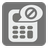Restricted Sum
Elementary
English FR JA RU UK

Our new calculator is censored and as such it does not accept certain words. You should try to trick by writing a program to calculate the sum of numbers.

Given a list of numbers, you should find the sum of these numbers. Your solution should not contain any of the banned words, even as a part of another word.

The list of banned words are as follows:

• sum
• import
• for
• while
• reduce

Input: A list of numbers.

Output: The sum of numbers.

Example:

```checkio([1, 2, 3]) == 6
checkio([2, 2, 2, 2, 2, 2]) == 12
```

How it is used: This task challenges your creativity to come up with a solution to fit this mission's specs!

Precondition: The small amount of data. Let's creativity win!